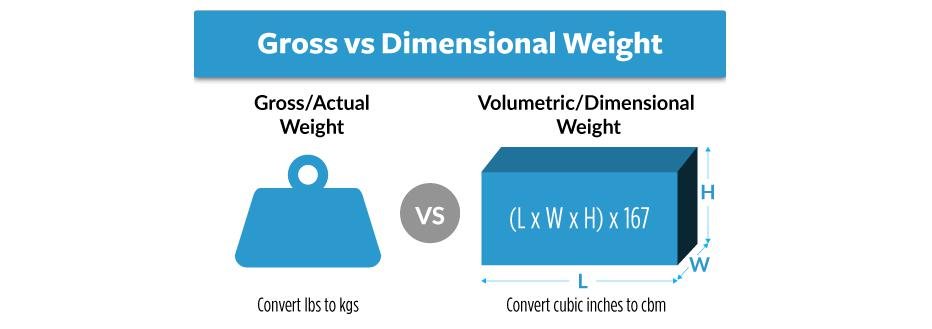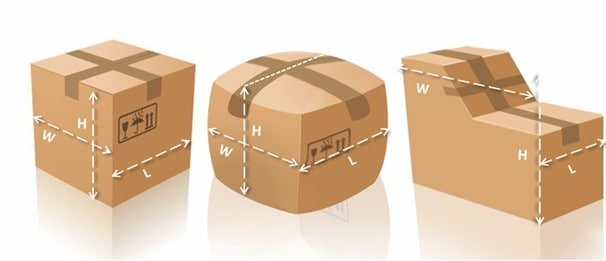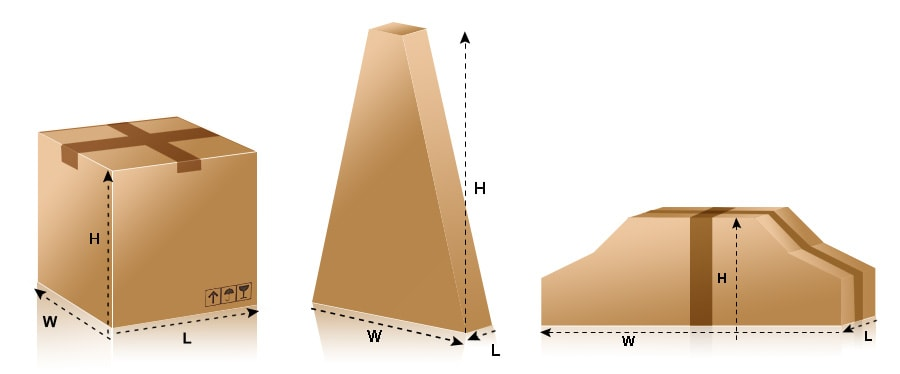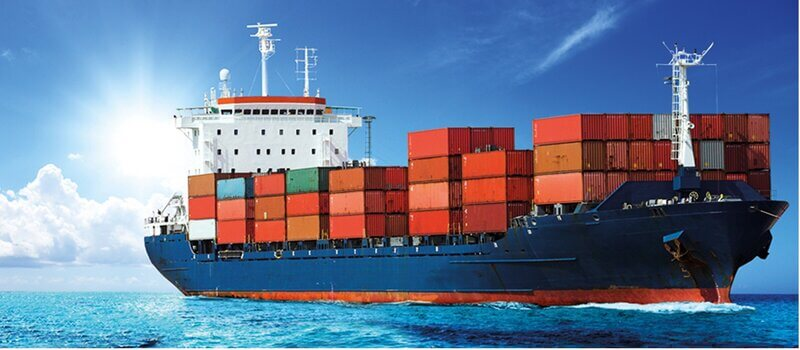# How to Calculate Chargeable Weight for Air & Sea Freight Shipments?

Why do freight forwarder companies not use the cargo’s gross or volumetric weight but rather the chargeable weight when calculating freight costs?

You see, some goods are heavy but take up minimal space, while others are very light but occupy a large volume in transportation.

Consider a shipment of cotton and steel. A cotton shipment will occupy more space compared to a steel shipment. However, a steel shipment will weigh more. Should both be charged using volumetric weight?They choose the higher value between the volumetric weight and the actual weight of the cargo. This is fair enough because cotton shipments will occupy more space while steel shipments will weigh more. In both cases, shippers will be choosing the measurement with greater value.

## What is the calculation formula for Chargeable Weight?

For those of you who simply want the formulas without a detailed explanation, here we go:

### Gross Weight VS Volumetric Weight

In international logistics, goods are categorized into lightweight goods and heavyweight goods. But what exactly are light goods, and what distinguishes them from heavy goods? To understand the definitions of lightweight and grave goods, we need to understand actual gross weight, volumetric weight, and chargeable weight.

### The Actual Weight:

Actual weight is the weight obtained from weighing (on a scale), including Gross Weight (G.W.) and Net Weight (N.W.). The most common measure is the actual gross weight.

In air cargo transportation, the actual gross weight is often compared to the calculated volumetric weight, and the higher of the two is used for calculation and charging.

### The Volume Weight

Volumetric weight, also known as dimensional weight, is calculated using a specific conversion factor or calculation formula based on the cargo volume.

In international freight transportation, the freight is calculated based on whichever value is greater between the actual weight and the volumetric weight of the goods.

Typically, lighter loads are charged based on dimensional weight, while heavier loads are charged based on actual weight.### Chargeable Weight

The Chargeable Weight, called C.W., calculates the weight for freight charges and other miscellaneous charges.

The chargeable weight is determined by either the actual gross weight or the volumetric weight, with the chargeable weight being greater. The formula for chargeable weight is chargeable weight = max(actual weight, volumetric weight).

## How do air freight company calculate the Chargeable Weight?

In air freight transportation, the calculation factor for volumetric weight is typically 1:167, meaning one cubic meter is approximately equal to 167 kilograms.

For instance, let’s consider an air cargo shipment with a gross weight of 95 kg and a volume of 1.2 cubic meters.

Calculated using the air transportation coefficient of 1:167, the volumetric weight for this cargo is 1.2 * 167 = 200.4 kg, which exceeds the actual gross weight of 95 kg.

As a result, this cargo, also known as soft or light goods (referred to as Light Weight Cargo, Light Cargo/Goods, Low-Density Cargo, or Measurement Cargo in English), will be charged based on volume and not actual gross weight.

In another scenario, if a shipment of air cargo has an actual gross weight of 560 kg and a volume of 1.5 CBM, calculated using the air transport coefficient of 1:167, the volumetric weight is 1.5 * 167 = 250.5 kg, which is less than the actual gross weight of 560 kg.

Consequently, this cargo is classified as heavy goods (known as Heavy Cargo/Goods or High-Density Cargo in English), and the airline companies will charge based on the actual gross weight, not the volumetric weight.

In summary, the volumetric weight is calculated using a specific conversion coefficient. The chargeable weight is then determined by comparing the volumetric weight with the actual weight, and the higher of the two is used for calculating charges.

### Regular items:

Length (cm) x width (cm) x height (cm) ÷ 6000 = volumetric weight (KG), ie 1 CBM ≈ 166.6666 KG.

### Irregular items:Irregularly Shaped Air freight Shipment

The longest (cm) x widest (cm) x highest (cm) ÷ 6000 = volumetric weight (KG), ie 1 CBM ≈ 166.666667 KG.

This is an internationally accepted algorithm.

In short, 1 cubic meter of weight greater than 166.67 kilograms is called heavy cargo, and less than 166.67 kilograms is called foam cargo in China.

Heavy goods are charged according to the actual gross weight, and the foamed goods are charged according to the volumetric weight.

### Air Freight Chargeable Weight Calcuation:

The gross weight of an air shipment can be higher in value compared to its volumetric weight. This makes it the chosen chargeable weight for the cargo as shown in the below example.

Shipment measurement:

• Length (l) = 80cm
• Width (w) = 20cm
• Height (h) = 45cm
• Gross Weight = 5Kgs

Step 1; Calculate the volume weight of the air freight shipment

Volume weight= length X width X height
= 80cm x 20cmx 45cm
= 11.4Kg

Step 2; Compare the gross weight with the volumetric weight

volumetric weight vs gross weight = 11.4 kgs vs 9.5kgs

Chargeable weight is the greater value which is 11.4 kgs.

## How does Express Freight calculate Chargeable Weight?

Express freight calculation methods:

There are three ways to measure the volume in practice:

### 1 Standard package measurement method:

The package is with a standard carton size,

The volume is calculated according to the length, height and width of the carton.

For example, DHL channel, package length 15cm, width 30cm, height 20cm,

Then the volume weight = (15 × 30 × 20) / 5000 = 1.8KG,

The actual weight is 1.2KG,

Then the parcel demensional weight calculated is 1.8KG.

The final DHL chargeable weight is 2KG.### 2 Surface package measurement method:

If the package is squeezed by the goods, the plane of the box expands and deforms,

Then calculate according to the longest side of the package.

For example, DHL channel, the actual length of the carton is 15cm, 30cm, and 20cm;

Due to the extrusion of the goods, the length is 18cm, 33cm, and 22cm after sealing.

Then the volume weight = (18 × 33 × 22) / 5000 = 2.7 KG,

The actual weight is 2KG,

Then the package calculates the shipping cost by the volume of 2.7KG.

The final billing weight is 3KG.

### 3 Irregular package measurement method:

Due to the actual size of the goods, it needs to be packaged into irregular packages.

Then the package is calculated according to the longest side and the volume is heavy.

Example: such as the UPS channel,

Irregular parcels have a maximum length of 15cm, a maximum length of 40cm, and a maximum height of 25cm.

Then the volume weight= (15 × 40 × 25) / 5000 = 3KG,

The actual weight is 2KG,

Finally, the package calculates the shipping cost by the volume weight of 3KG.

However, what customers often overlook is the design of the box itself. The size indicated on the box is the inner diameter of the box, it does not contain the thickness of the paper, but actual shipment is measured by the outer dimensions of the box.

### Notices:

1. CBM is the abbreviation for Cubic Meter, representing a cubic meter.

2. This algorithm, length (cm) × width (cm) × height (cm) ÷ 5000, is utilized only by express companies (DHL, FedEx, TNT, UPS, Aramex) to calculate the volumetric weight.

3. To optimize an airplane’s space and load capacity, the loading of heavy and light cargo is typically carefully balanced. Air cargo loading is a technical activity. When done effectively, it fully utilizes the limited space of the aircraft, potentially resulting in increased profit.

Overloading the plane with too many heavy goods can result in wasted space (exceeding weight capacity without occupying the room). Conversely, excessive cargo can lead to wasted load (reaching the maximum weight without utilizing all available space).

## Sea Freight Chargeable Weight CalculationThe categorization of goods as heavy or light in sea freight is much simpler compared to air transportation. China’s sea freight consolidation business primarily categorizes goods based on the standard of 1 cubic meter equal to 1 ton (1 CBM = 1000 KG).

Heavy goods are rare in less-than-container-load (LCL) ocean freight; most are light goods. Shipping LCL is calculated based on freight volume, differing fundamentally from air transport, which is based on the weight of the freight, making the process simpler. Many individuals involved in shipping may have yet to hear of light goods and heavy goods since they are not commonly used terms.

Regarding ship stowage, any cargo with a cargo stowage factor less than the ship’s capacity factor is labeled as Heavy Weight Cargo. In contrast, any cargo with a cargo stowage factor more significant than the ship’s tank capacity is termed Measurement Cargo/Light Goods.

According to freight calculation and international shipping business practices, any cargo with a stowage factor of less than 1.1328 m3/ton or 40 cubic feet/ton is categorized as heavy cargo. Cargo with a stowage factor greater than 1.1328 m3/ton or 40 cubic feet per ton is called light goods or foam goods.

Classifying goods into heavy and light is closely tied to storage, transportation, storage, and billing. Carriers or freight forwarder companies differentiate between heavy and light goods/foam goods based on specific standards.

If you require assistance with moving your cargo, whether via air or sea, we are more than happy to help. Contact us now and send a message for a quote for your air or sea freight from China.”